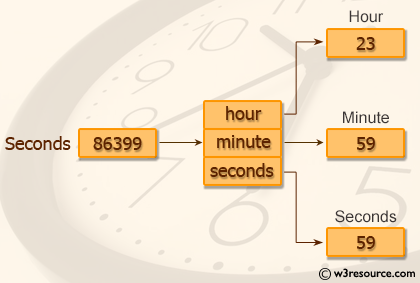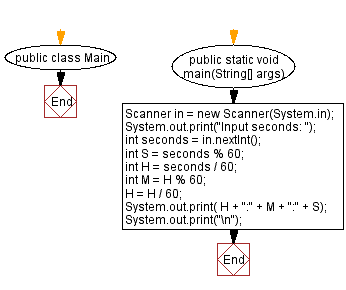﻿ Java exercises: Convert seconds to hour, minute and seconds - w3resource# Java Exercises: Convert seconds to hour, minute and seconds

## Java Basic: Exercise-55 with Solution

Write a Java program to convert seconds to hour, minute and seconds.

Pictorial Presentation:Sample Data:
Input seconds: 86399
23:59:59

Sample Solution:

Java Code:

``````import java.util.*;
public class Exercise55 {
public static void main(String[] args)
{
Scanner in = new Scanner(System.in);
System.out.print("Input seconds: ");
int seconds = in.nextInt();
int p1 = seconds % 60;
int p2 = seconds / 60;
int p3 = p2 % 60;
p2 = p2 / 60;
System.out.print( p2 + ":" + p3 + ":" + p1);
System.out.print("\n");
}
}
```
```

Sample Output:

```Input seconds: 86399
23:59:59
```

Pictorial Presentation:Flowchart:Java Code Editor:

What is the difficulty level of this exercise?

Test your Programming skills with w3resource's quiz.

﻿

## Java: Tips of the Day

countOccurrences

Counts the occurrences of a value in an array.

Use Arrays.stream().filter().count() to count total number of values that equals the specified value.

```public static long countOccurrences(int[] numbers, int value) {
return Arrays.stream(numbers)
.filter(number -> number == value)
.count();
}
```

Ref: https://bit.ly/3kCAgLb